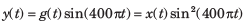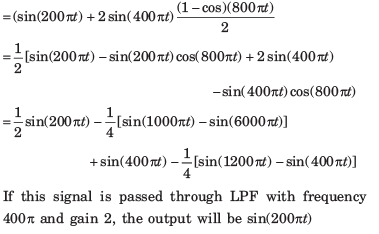Courses

# Amplitude Modulation - MCQ Test

## 20 Questions MCQ Test GATE ECE (Electronics) 2022 Mock Test Series | Amplitude Modulation - MCQ Test

Description
This mock test of Amplitude Modulation - MCQ Test for Electronics and Communication Engineering (ECE) helps you for every Electronics and Communication Engineering (ECE) entrance exam. This contains 20 Multiple Choice Questions for Electronics and Communication Engineering (ECE) Amplitude Modulation - MCQ Test (mcq) to study with solutions a complete question bank. The solved questions answers in this Amplitude Modulation - MCQ Test quiz give you a good mix of easy questions and tough questions. Electronics and Communication Engineering (ECE) students definitely take this Amplitude Modulation - MCQ Test exercise for a better result in the exam. You can find other Amplitude Modulation - MCQ Test extra questions, long questions & short questions for Electronics and Communication Engineering (ECE) on EduRev as well by searching above.
QUESTION: 1

### A carrier is simultaneously modulated by two sine waves with modulation indices of 0.4 and 0.3. The resultant modulation index will be

Solution: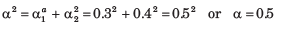QUESTION: 2

### In a DSB-SC system with 100% modulation, the power saving is

Solution:

In previous solution Pc = 2/3P. If carrier is suppressed then 2/3 P or 66% power will be saved.

QUESTION: 3

### A 10 kW carrier is sinusoidally modulated by two carriers corresponding to a modulation index of 30% and 40% respectively. The total radiated power(is

Solution: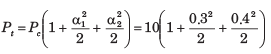= 11.25 kW

QUESTION: 4

In amplitude modulation, the modulation envelope has a peak value which is double the unmodulated carrier value. What is the value of the modulation index ?

Solution: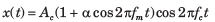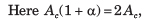Thus α = 1, therefor modulation index is 1 or 100% modulation.

QUESTION: 5

If the modulation index of an AM wave is changed from 0 to 1, the transmitted power

Solution: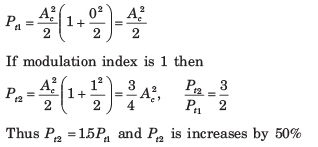QUESTION: 6

A diode detector has a load of 1 kΩ shunted by a 10000 pF capacitor. The diode has a forward resistance of 1 Ω. The maximum permissible depth of modulation,so as to avoid diagonal clipping, with modulating signal frequency fo 10 kHz will be

Solution: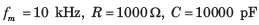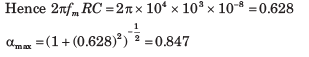QUESTION: 7

An AM signal is detected using an envelop detector.The carrier frequency and modulating signal frequency are 1 MHz and 2 kHz respectively. An appropriate value for the time constant of the envelope detector is.

Solution: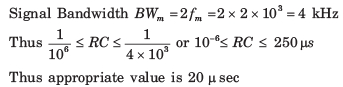QUESTION: 8

A 1 MHz sinusoidal carrier is amplitude modulated by a symmetrical square wave of period 100 μ sec. Which of the following frequencies will NOT be present in the modulated signal?

Solution: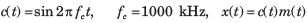Expressing square wave as modulating signal m(t)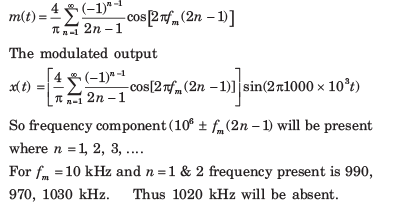QUESTION: 9

For an AM signal, the bandwidth is 10 kHz and the highest frequency component present is 705 kHz. The carrier frequency used for this AM signal is

Solution: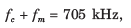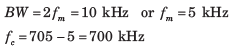QUESTION: 10

A message signal m(t) = sinct + sinc2(t)  modulates the carrier signal (t) = Acos2πfct . The bandwidth of the modulated signal is

Solution: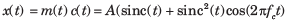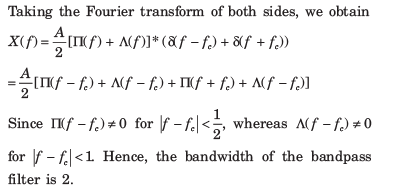QUESTION: 11

Consider the system shown in figure . The average value of m(t) is zero and maximum value of
|m(t)| is M. The square-law device is defined by y(t) = 4x(t) +10 x(t).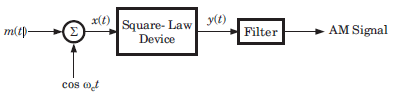Que: The value of M, required to produce modulation index of 0.8, is

Solution: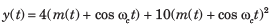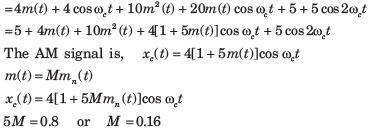QUESTION: 12

Consider the system shown in figure . The average value of m(t) is zero and maximum value of
|m(t)| is M. The square-law device is defined by y(t) = 4x(t) +10 x(t).Let W be the bandwidth of message signal m(t). AM signal would be recovered if

Solution: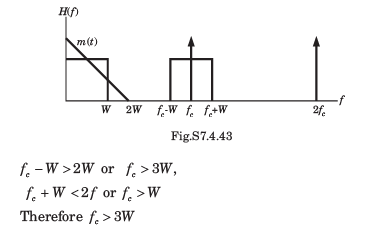QUESTION: 13

A super heterodyne receiver is designed to receive transmitted signals between 5 and 10 MHz. High-side tuning is to be used. The tuning range of the local oscillator for IF frequency 500 kHz would be

Solution:

Since High-side tuning is used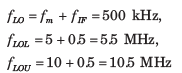QUESTION: 14

A super heterodyne receiver uses an IF frequency of 455 kHz. The receiver is tuned to a transmitter having a carrier frequency of 2400 kHz. High-side tuning is to be used. The image frequency will be

Solution: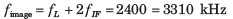QUESTION: 15

In the circuit shown in fig, the transformers are center tapped and the diodes are connected as shown in a bridge. Between the terminals 1 and 2 an a.c. voltage source of frequency 400 Hz is connected. Another a.c. voltage of 1.0 MHz is connected between 3 and 4. The output between 5 and 6 contains components atSolution:

The given circuit is a ring modulator. The output is DSB-SC signal. So it will contain m(t) cos( nwct) where n = 1,2, 3...... Therefore there will be only (1 MHz +- 400 Hz) frequency component.

QUESTION: 16

A superheterodyne receiver is to operate in the frequency range 550 kHz-1650 kHz, with the intermediate frequency of 450 kHz. Let R = cmax / cmin   denote the required capacitance ratio of the local oscillator and I denote the image frequency (in kHz) of the incoming signal. If the receiver is tuned to 700 kHz, then

Solution:

fmax = 1650 + 450 = 2100 kHz

fmin = 550 + 450 = 1000 kHz.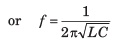QUESTION: 17

Consider a system shown in Figure . Let X( f ) and Y( f ) denote the Fourier transforms of x(t) and y(t) respectively. The ideal HPF has the cutoff frequency 10 kHz. The positive frequencies where Y( f ) has spectral peaks are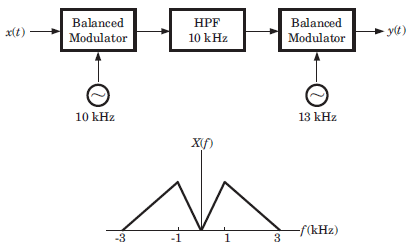Solution:

Since X (f) has spectral peak at 1 kHz so at the output of first modulator spectral peak will be at (10 + 1) kHz and (10  1) kHz. After passing the HPF frequency component of 11 kHz will remain. The output of 2nd modulator will be (13 +11) kHz. So Y (f) has spectral peak at 2 kHz and 24 kHz.

QUESTION: 18

In fig.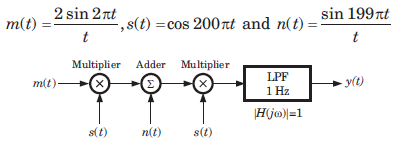The output y(t) will be

Solution:

m(t).s(t) = y1(t)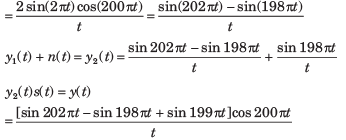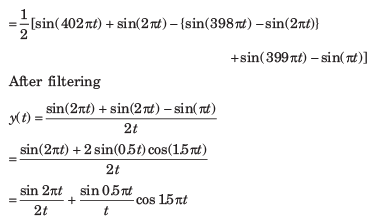QUESTION: 19

What is the carrier frequency in an AM wave when its highest frequency component is 850Hz and the bandwidth of the signal is 50Hz?

Solution:

Upper frequency = 850Hz
Bandwidth = 50Hz
Therefore lower Frequency = 850 - 50= 800 Hz
Carrier Frequency = (850-800)/2
= 825 Hz

QUESTION: 20

Suppose we wish to transmit the signal x(t) = sin 200πt + 2 sin 400πt using a modulation that create the signal g(t) = x(t) sin 400πt. If the product g(t) sin 400πt is passed through an ideal LPF with cutoff frequency 400π and pass band gain of 2, the signal obtained at the output of the LPF is

Solution: